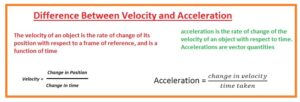Hello guys, I hope you all are doing great. In today’s tutorial, we will have a look at Difference Between Velocity and Acceleration. There are 2 most important parameter velocity and acceleration related to the motion of different bodies. The main difference between these two parameters is that velocity changes in the position of a body in a specific direction in unit time. While acceleration is the change in velocity in a specific direction per unit time.

In today’s post, we will have a detailed look at different parameters of velocity and acceleration and find differences. So let’s get started with Difference Between Velocity and Acceleration.

#### Difference Between Velocity and Acceleration

Velocity

• The variation in the position of a body in unit time is called velocity.
• Its measuring formula is meter per second.
• The rate of change in the position of the body with time is called velocity.
• It is equivalent to the body speed feature having direction and movement.
• It mostly used in the science field of kinematics.
• It is a physical term has velocity tan magnitude.
• Its unit is m/s
• The velocity measured between two objects called relative velocity.
• Its dimension is LT−1
• The moving body has zero value of speed
• Change in velocity called acceleration
• Speed measured at any instant of moving object called instantaneous speed
• The product of mass and velocity equal to the momentum.
• Speed is related to the displacement of the body.
• It also known as a change in displacement of body.## Calculation of Velocity

Velocity can be measured by dividing the displacement of anybody by the time taken. The formula for calculating velocity is:

• v = s/t

where: v = velocity s = displacement t = time taken

### What is Speed

• Speed is denoted with V and is the magnitude of variation in the position of anybody and it is a scalar term.
• The time duration in which the distance covered through body parted in different parts is called average speed.
• The system international unit used for speed is meter per second. In some cases, kilometers per hour is used

### Difference between Speed and Velocity

• Speed tells about the motion of an object and the quantity of a moving object while velocity tells about the speed quantity and motion of direction.
• Let us tale an example car moving with a speed of thirty kilometers per second and its direction is toward south then its speed is given

### Tangential Speed

• Tangential speed is caused due to the body moving in a circle.In this case, the direction of motion of the object lies at the tangent to the circle so it is called tangential speed

Acceleration

• The rate of change of velocity is called acceleration.
• The direction of acceleration is found through the total force acting at the body
• The magnitude of the acceleration is explained through the use of the second law of newton
• Its meauring unit is m/s^2.
• The product of mass and acceleration is equal to the force.
• It is related to the velocity of the body.

## Calculation of Acceleration

The formula for the calculation of acceleration is given here.

• a = (v-u)/t

where: a = acceleration v = final velocity u = initial velocity t = time taken

Velocity Acceleration
rate of change of an object’s position with respect to time is known as velocity.  rate of change of velocity body  with respect to time is called acceleration
Velocity is a vector quantity, which has both magnitude and direction. Acceleration is a vector quantity, that also has magnitude and direction.
velocity direction defines the direction in which the body is moving. acceleration direction indicates the direction in which the velocity of the body is varying
Velocity magnitude defines the speed of the body at which it moving Its magnitude defines the rate at which the velocity of the object is varying
The measuring unit is meters per second (m/s). Its measuring unit is meters per second squared (m/s^2).

### What is Uniform Acceleration

• The acceleration in which the velocity of any moving body varies through equal quantity in  given time is called uniform acceleration.

That is a detailed post about the difference between velocity and acceleration if you have any query ask in the comments. Thanks for reading. Have a good day.